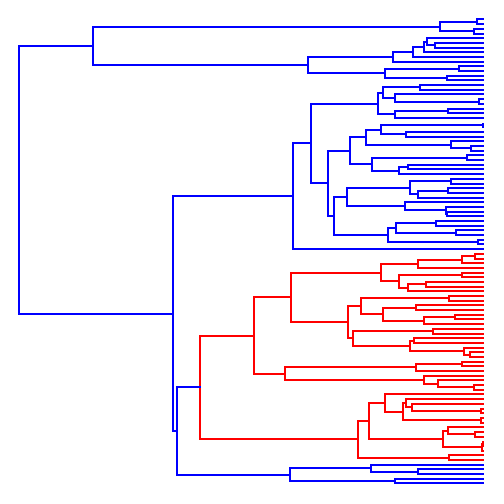## Wednesday, February 7, 2018

### Update to Mk simulators for multiple simulations

I just pushed updates for the relatively new phytools functions `sim.Mk` and `sim.multiMk` that permit the functions to simulate more than one character at a time.

Though previously this would have been trivial to script using (for instance) a single `replicate` call, the advantage gained by adding it to the function directly is that the different transition matrices for each edge (or edge segment, in the case of `fit.multiMk`) only need to be computed once by the function instead of separately for each simulation.

Here is an example of the the speed-up:

``````library(phytools)
packageVersion("phytools")
``````
``````##  '0.6.55'
``````
``````tree<-pbtree(n=100,scale=2)
``````
``````Q<-matrix(c(-1,1,0,1,-2,1,0,1,-1),3,3,byrow=T,
dimnames=list(0:2,0:2))
Q
``````
``````##    0  1  2
## 0 -1  1  0
## 1  1 -2  1
## 2  0  1 -1
``````
``````## old way:
system.time(X<-replicate(100,sim.Mk(tree,Q)))
``````
``````##    user  system elapsed
##    3.14    0.00    3.14
``````
``````## new way:
system.time(X<-sim.Mk(tree,Q,nsim=100))
``````
``````##    user  system elapsed
##    0.32    0.00    0.31
``````

Much faster.

For fun, let's take our second set of simulations and fit an ordered, single-rate Mk model to each of them. (This is the model we used for simulation.)

``````ordered<-matrix(c(0,1,0,1,0,1,0,1,0),3,3)
fits<-apply(X,2,fitMk,tree=tree,model=ordered)
q<-sapply(fits,function(x) x\$rates)
mean(q)
``````
``````##  1.037991
``````

This should be around 1.0 - the value we used for simulation.

Now let's do the same for a heterogeneous rate process:

``````Q<-setNames(list(
matrix(c(-0.5,0.5,0,0.5,-1,0.5,0,0.5,-0.5),3,3,dimnames=list(0:2,0:2)),
matrix(c(-2,2,0,2,-4,2,0,2,-2),3,3,dimnames=list(0:2,0:2))),c("a","b"))
Q
``````
``````## \$a
##      0    1    2
## 0 -0.5  0.5  0.0
## 1  0.5 -1.0  0.5
## 2  0.0  0.5 -0.5
##
## \$b
##    0  1  2
## 0 -2  2  0
## 1  2 -4  2
## 2  0  2 -2
``````
``````tree<-paintSubTree(tree,108,"b","a")
cols<-setNames(c("blue","red"),c("a","b"))
plot(tree,cols,ftype="off")
````````````X<-sim.multiMk(tree,Q,nsim=100)
fits<-apply(X,2,fitmultiMk,tree=tree,model=ordered)
q<-t(sapply(fits,function(x) x\$rates))
colMeans(q)
``````
``````##  0.5427954 2.1405881
``````

Again, hopefully these are similar to 0.5 and 2.0.